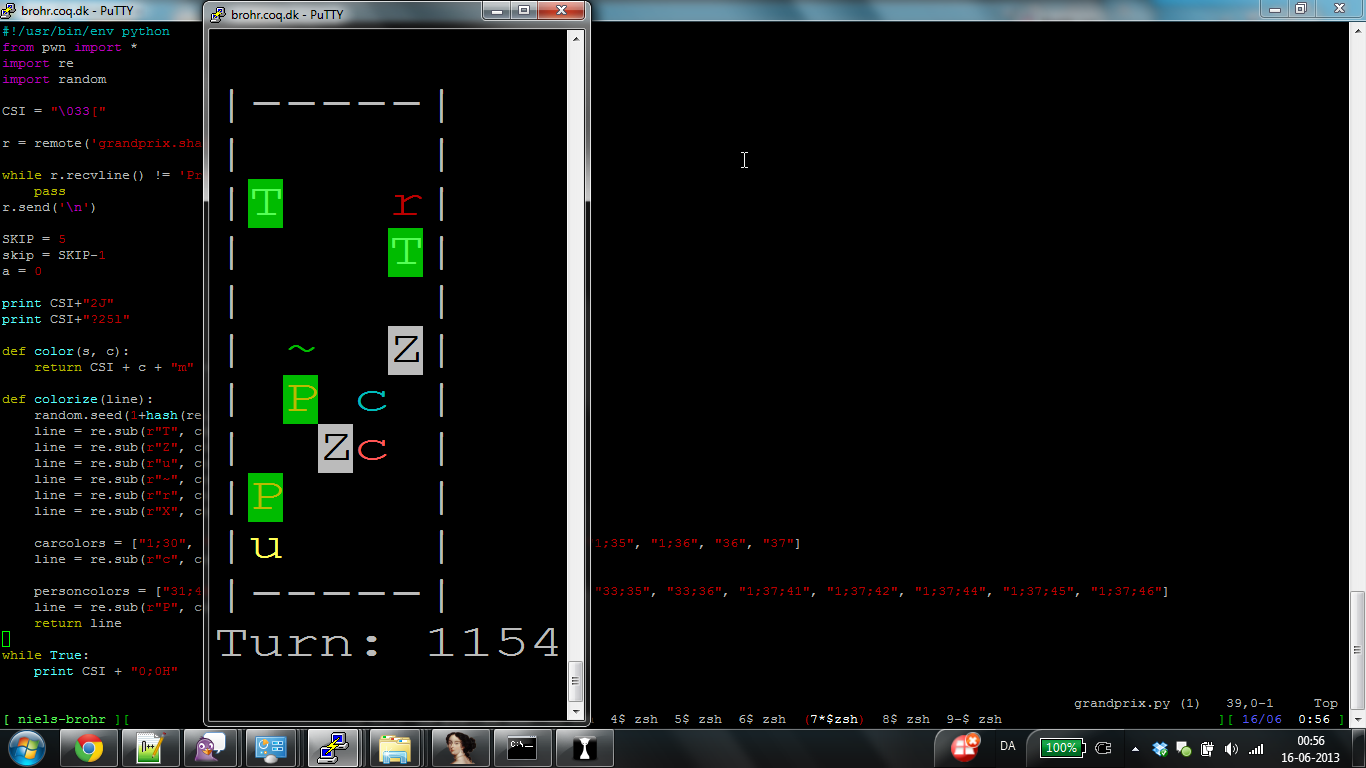# grandprix - DEFCON CTF quals 2013

Posted by sebbe on 20 Jun 2013

In the 3 point challenge in the OMGACM ("guerilla programming") track, we were given a server to connect to. Connecting to this service, it quickly became clear, that the point of the challenge was to write a program to maneuver a car through a simple ASCII racing track, while avoiding obstacles on the road (zebras, cars, ...).

To do this, we simply started at the location of our own car and did a depth-first search up to the top of the screen. As soon as we had a viable path, we drive 5 spaces along this path, and process the next board.

In order to make it a bit more interesting and fun, we also added some pretty colors to make it more interesting to watch on rather slow servers.The final script we ended up with, pretty colors and all, was this:

``````#!/usr/bin/env python
from pwn import *
import re
import random

CSI = "\033["

r = remote('grandprix3.shallweplayaga.me',2038)

pass
r.send('\n')

SKIP = 5
skip = SKIP-1
a = 0

print CSI+"2J"
print CSI+"?25l"

def color(s, c):
return CSI + c + "m" + s + CSI + "0m"

def colorize(line):
random.seed(1+hash(re.sub(r"u", " ", line)))
line = re.sub(r"T", color("T", "1;32;42"), line)
line = re.sub(r"Z", color("Z", "30;47"), line)
line = re.sub(r"u", color(r"u", "1;33"), line)
line = re.sub(r"~", color(r"~", "32"), line)
line = re.sub(r"r", color(r"r", "31"), line)
line = re.sub(r"X", color(r"X", "1;41;37"), line)

carcolors = ["1;30", "1;31", "32", "1;32", "33", "34", "1;34", "35", "1;35", "1;36", "36", "37"]
line = re.sub(r"c", color(r"c", random.choice(carcolors)), line)

personcolors = ["31;44", "31;43","31;46",  "33;42", "33;41", "33;44", "33;35", "33;36", "1;37;41", "1;37;42", "1;37;44", "1;37;45", "1;37;46"]
line = re.sub(r"P", color(r"P", random.choice(personcolors)), line)
return line

while True:
print CSI + "0;0H"

a += 1
s = []
cnt = 0
while cnt < 11:
line = r.recvline().strip()
if len(line) == 0: continue
cnt += 1
print colorize(line)
s.append(line)
if line == 'Too slow!':
print "Got 'Too slow!' after", a, "turns"
exit(0)

print "Turn: %d" % a

skip = (skip+1) % SKIP
if skip > 0: continue

ourpos = len(s)-2
index = s[ourpos].find('u')

def do_search(row,prev_pos,path,paths):
if row == 1:
paths.append(path)
raise None

cur = s[row]

if cur[prev_pos] == ' ' or cur[prev_pos] == '=':
new_path = path + ' '
do_search(row-1, prev_pos, new_path, paths)
if prev_pos > 1 and (cur[prev_pos-1] == ' ' or cur[prev_pos-1] == '='):
new_path = path + 'l'
do_search(row-1, prev_pos-1, new_path, paths)
if prev_pos < 5 and (cur[prev_pos+1] == ' ' or cur[prev_pos+1] == '='):
new_path = path + 'r'
do_search(row-1, prev_pos+1, new_path, paths)

ps = []
try:
do_search(ourpos-1, index, '', ps)
except:
pass

apath = ps
msg = ""
for i in range(SKIP):
msg += "%s\n" % apath[i]
r.send(msg)
``````